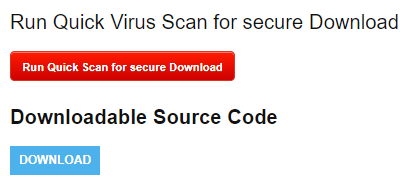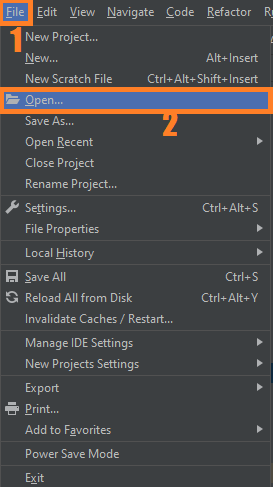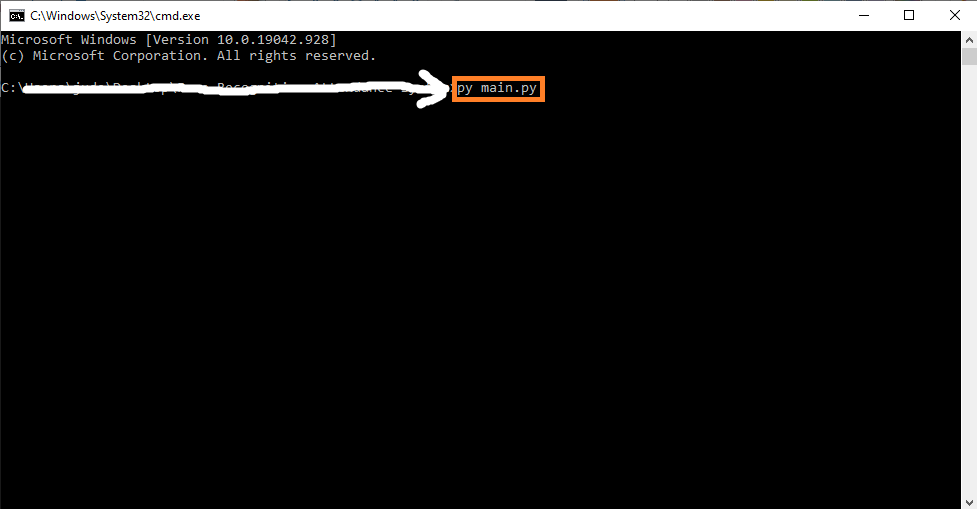# Cafe Management System In Python With Source Code

## Cafe Management System In Python With Source Code

The Cafe Management System In Python was developed using Python Programming, This Cafe Management System Project In Python is a simple GUI based application which is very easy to understand and use. It uses Tkinter module for the GUI.

Talking about the application, the user just has to select among the food and drinks items, enter the quantity and click on the total button to view the total price. This simple application also displays the total prices of each item without any tax and extra charges.

A Cafe Management System Using Python user can view the total receipt of their items which displays receipt number and number of their food/drinks items with the total amount. Here, the total bill of the customer includes tax and service charges too.

The design is so simple that the user won’t find any difficulties while working on it. In order to run the project, you must have installed Python and on your PC. This is a simple cafe billing system application for beginners.

By the way if you are new to python programming and you don’t know what would be the the Python IDE to use, I have here a list of Best Python IDE for Windows, Linux, Mac OS that will suit for you. I also have here How to Download and Install Latest Version of Python on Windows.

To start executing Cafe Management System In Python With Source Code, make sure that you have installed Python 3.9 and PyCharm in your computer.

## Cafe Management System In Python With Source Code : Steps on how to run the project

Time needed: 5 minutes.

These are the steps on how to run Cafe Management System In Python With Source Code

First, download the given source code below and unzip the source code.• Step 2: Import the project to your PyCharm IDE.• Step 3: Run the project.

last, run the project with the command “py main.py”## Installed Libraries

```from tkinter import*
import random
import time```

## Complete Source Code

```from tkinter import*
import random
import time

root = Tk()
root.geometry("890x580+0+0")
root.title("SIMPLE CAFE BILLING SYSTEM")

Tops = Frame(root,bg="white",width = 1600,height=50,relief=SUNKEN)
Tops.pack(side=TOP)

f1 = Frame(root,width = 900,height=700,relief=SUNKEN)
f1.pack(side=LEFT)

f2 = Frame(root ,width = 400,height=700,relief=SUNKEN)
f2.pack(side=RIGHT)
#------------------TIME--------------
localtime=time.asctime(time.localtime(time.time()))
#-----------------INFO TOP------------
lblinfo = Label(Tops, font=( 'aria' ,30, 'bold' ),text="SIMPLE CAFE BILLING MANAGEMENT SYSTEM",fg="Black",bd=10,anchor='w')
lblinfo.grid(row=0,column=0)
lblinfo = Label(Tops, font=( 'aria' ,20, ),text=localtime,fg="steel blue",anchor=W)
lblinfo.grid(row=1,column=0)

def Ref():
x=random.randint(12980, 50876)
randomRef = str(x)
rand.set(randomRef)

cof =float(Fries.get())
colfries= float(Largefries.get())
cob= float(Burger.get())
cofi= float(Filet.get())
cochee= float(Cheese_burger.get())
codr= float(Drinks.get())

costoffries = cof*25
costoflargefries = colfries*40
costofburger = cob*35
costoffilet = cofi*50
costofcheeseburger = cochee*30
costofdrinks = codr*35

costofmeal = "Rs.",str('%.2f'% (costoffries +  costoflargefries + costofburger + costoffilet + costofcheeseburger + costofdrinks))
PayTax=((costoffries +  costoflargefries + costofburger + costoffilet +  costofcheeseburger + costofdrinks)*0.33)
Totalcost=(costoffries +  costoflargefries + costofburger + costoffilet  + costofcheeseburger + costofdrinks)
Ser_Charge=((costoffries +  costoflargefries + costofburger + costoffilet + costofcheeseburger + costofdrinks)/99)
Service="Rs.",str('%.2f'% Ser_Charge)
OverAllCost="Rs.",str( PayTax + Totalcost + Ser_Charge)
PaidTax="Rs.",str('%.2f'% PayTax)

Service_Charge.set(Service)
cost.set(costofmeal)
Tax.set(PaidTax)
Subtotal.set(costofmeal)
Total.set(OverAllCost)

def qexit():
root.destroy()

def reset():
rand.set("")
Fries.set("")
Largefries.set("")
Burger.set("")
Filet.set("")
Subtotal.set("")
Total.set("")
Service_Charge.set("")
Drinks.set("")
Tax.set("")
cost.set("")
Cheese_burger.set("")

#---------------------------------------------------------------------------------------
rand = StringVar()
Fries = StringVar()
Largefries = StringVar()
Burger = StringVar()
Filet = StringVar()
Subtotal = StringVar()
Total = StringVar()
Service_Charge = StringVar()
Drinks = StringVar()
Tax = StringVar()
cost = StringVar()
Cheese_burger = StringVar()

lblreference = Label(f1, font=( 'aria' ,16, 'bold' ),text="Order No.",fg="green",bd=20,anchor='w')
lblreference.grid(row=0,column=0)
txtreference = Entry(f1,font=('ariel' ,16,'bold'), textvariable=rand , bd=6,insertwidth=6,bg="blue" ,justify='right')
txtreference.grid(row=0,column=1)

lblfries = Label(f1, font=( 'aria' ,16, 'bold' ),text=" French Fries ",fg="blue",bd=10,anchor='w')
lblfries.grid(row=2,column=0)
txtfries = Entry(f1,font=('ariel' ,16,'bold'), textvariable=Fries , bd=6,insertwidth=4,bg="gray" ,justify='right')
txtfries.grid(row=2,column=1)

lblLargefries = Label(f1, font=( 'aria' ,16, 'bold' ),text="Lunch ",fg="blue",bd=10,anchor='w')
lblLargefries.grid(row=3,column=0)
txtLargefries = Entry(f1,font=('ariel' ,16,'bold'), textvariable=Largefries , bd=6,insertwidth=4,bg="gray" ,justify='right')
txtLargefries.grid(row=3,column=1)

lblburger = Label(f1, font=( 'aria' ,16, 'bold' ),text="Burger ",fg="blue",bd=10,anchor='w')
lblburger.grid(row=4,column=0)
txtburger = Entry(f1,font=('ariel' ,16,'bold'), textvariable=Burger , bd=6,insertwidth=4,bg="gray" ,justify='right')
txtburger.grid(row=4,column=1)

lblFilet = Label(f1, font=( 'aria' ,16, 'bold' ),text="Pizza ",fg="blue",bd=10,anchor='w')
lblFilet.grid(row=5,column=0)
txtFilet = Entry(f1,font=('ariel' ,16,'bold'), textvariable=Filet , bd=6,insertwidth=4,bg="gray" ,justify='right')
txtFilet.grid(row=5,column=1)

lblCheese_burger = Label(f1, font=( 'aria' ,16, 'bold' ),text="Cheese burger",fg="blue",bd=10,anchor='w')
lblCheese_burger.grid(row=6,column=0)
txtCheese_burger = Entry(f1,font=('ariel' ,16,'bold'), textvariable=Cheese_burger , bd=6,insertwidth=4,bg="gray" ,justify='right')
txtCheese_burger.grid(row=6,column=1)

#--------------------------------------------------------------------------------------
lblDrinks = Label(f1, font=( 'aria' ,16, 'bold' ),text="Drinks",fg="blue",bd=10,anchor='w')
lblDrinks.grid(row=1,column=0)
txtDrinks = Entry(f1,font=('ariel' ,16,'bold'), textvariable=Drinks , bd=6,insertwidth=4,bg="gray" ,justify='right')
txtDrinks.grid(row=1,column=1)

lblcost = Label(f1, font=( 'aria' ,16, 'bold' ),text="Cost",fg="black",bd=10,anchor='w')
lblcost.grid(row=2,column=2)
txtcost = Entry(f1,font=('ariel' ,16,'bold'), textvariable=cost , bd=6,insertwidth=4,bg="white" ,justify='right')
txtcost.grid(row=2,column=3)

lblService_Charge = Label(f1, font=( 'aria' ,16, 'bold' ),text="Service Charge",fg="black",bd=10,anchor='w')
lblService_Charge.grid(row=3,column=2)
txtService_Charge = Entry(f1,font=('ariel' ,16,'bold'), textvariable=Service_Charge , bd=6,insertwidth=4,bg="white" ,justify='right')
txtService_Charge.grid(row=3,column=3)

lblTax = Label(f1, font=( 'aria' ,16, 'bold' ),text="Tax",fg="black",bd=10,anchor='w')
lblTax.grid(row=4,column=2)
txtTax = Entry(f1,font=('ariel' ,16,'bold'), textvariable=Tax , bd=6,insertwidth=4,bg="white" ,justify='right')
txtTax.grid(row=4,column=3)

lblSubtotal = Label(f1, font=( 'aria' ,16, 'bold' ),text="Subtotal",fg="black",bd=10,anchor='w')
lblSubtotal.grid(row=5,column=2)
txtSubtotal = Entry(f1,font=('ariel' ,16,'bold'), textvariable=Subtotal , bd=6,insertwidth=4,bg="white" ,justify='right')
txtSubtotal.grid(row=5,column=3)

lblTotal = Label(f1, font=( 'aria' ,16, 'bold' ),text="Total",fg="green",bd=10,anchor='w')
lblTotal.grid(row=6,column=2)
txtTotal = Entry(f1,font=('ariel' ,16,'bold'), textvariable=Total , bd=6,insertwidth=4,bg="grey" ,justify='right')
txtTotal.grid(row=6,column=3)

#-----------------------------------------buttons------------------------------------------
lblTotal = Label(f1,text="---------------------",fg="white")
lblTotal.grid(row=7,columnspan=3)

btnTotal.grid(row=8, column=1)

btnreset.grid(row=8, column=2)

btnexit.grid(row=8, column=3)

def price():
roo = Tk()
roo.geometry("600x220+0+0")
roo.title("Price List")
lblinfo = Label(roo, font=('aria', 15, 'bold'), text="ITEM", fg="black", bd=5)
lblinfo.grid(row=0, column=0)
lblinfo = Label(roo, font=('aria', 15,'bold'), text="_____________", fg="white", anchor=W)
lblinfo.grid(row=0, column=2)
lblinfo = Label(roo, font=('aria', 15, 'bold'), text="PRICE", fg="black", anchor=W)
lblinfo.grid(row=0, column=3)
lblinfo = Label(roo, font=('aria', 15, 'bold'), text="French Fries", fg="steel blue", anchor=W)
lblinfo.grid(row=1, column=0)
lblinfo = Label(roo, font=('aria', 15, 'bold'), text="25", fg="steel blue", anchor=W)
lblinfo.grid(row=1, column=3)
lblinfo = Label(roo, font=('aria', 15, 'bold'), text="Lunch ", fg="steel blue", anchor=W)
lblinfo.grid(row=2, column=0)
lblinfo = Label(roo, font=('aria', 15, 'bold'), text="40", fg="steel blue", anchor=W)
lblinfo.grid(row=2, column=3)
lblinfo = Label(roo, font=('aria', 15, 'bold'), text="Burger ", fg="steel blue", anchor=W)
lblinfo.grid(row=3, column=0)
lblinfo = Label(roo, font=('aria', 15, 'bold'), text="35", fg="steel blue", anchor=W)
lblinfo.grid(row=3, column=3)
lblinfo = Label(roo, font=('aria', 15, 'bold'), text="Pizza ", fg="steel blue", anchor=W)
lblinfo.grid(row=4, column=0)
lblinfo = Label(roo, font=('aria', 15, 'bold'), text="50", fg="steel blue", anchor=W)
lblinfo.grid(row=4, column=3)
lblinfo = Label(roo, font=('aria', 15, 'bold'), text="Cheese Burger", fg="steel blue", anchor=W)
lblinfo.grid(row=5, column=0)
lblinfo = Label(roo, font=('aria', 15, 'bold'), text="30", fg="steel blue", anchor=W)
lblinfo.grid(row=5, column=3)
lblinfo = Label(roo, font=('aria', 15, 'bold'), text="Drinks", fg="steel blue", anchor=W)
lblinfo.grid(row=6, column=0)
lblinfo = Label(roo, font=('aria', 15, 'bold'), text="35", fg="steel blue", anchor=W)
lblinfo.grid(row=6, column=3)

roo.mainloop()

btnprice.grid(row=8, column=0)

root.mainloop()```